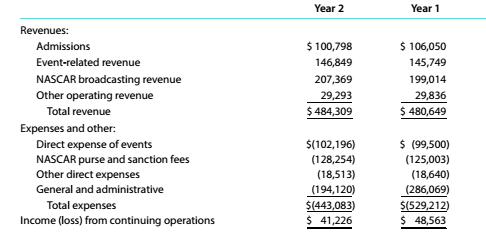Chapter 9, Problem 9.2E

Chapter
Section
Textbook Problem

Vertical analysis of income statementThe following comparative income statement (in thousands of dollars) for two recent years was adapted from the annual report of Speedway Motorsports. Inc. (TRK), owner and operator of several major motor speedways, such as the Atlanta, Bristol, Charlotte, Texas, and Las Vegas Motor Speedways.a. Prepare a comparative income statement for Years 1 and 2 in vertical form, staling each item as a percent of revenues. Round to one decimal place. b. Comment on the significant changes.

To determine

Concept Introduction:

Vertical Analysis:

Vertical Analysis is used to analyses the % of items in statement for a particular period as the % of total amount. Vertical Analysis is done for particular period for different items. For example analysis of % of assets as % of Total assets for a particular year is done using the Vertical analysis.

Requirement-a:

To Prepare:

A comparative income statement using Vertical analysis

Explanation

A comparative income statement using Vertical analysis is prepared as follows:

 Comparative income statement using Vertical analysis 20Y8 20Y8 \$ Common Size % \$ Common Size % A B = A / Sales C D = C / Sales Sales \$ 900,000 100.0% \$ 725,000 100.0% Cost of Goods sold \$(558,000) -62...
To determine

Concept Introduction:

Vertical Analysis:

Vertical Analysis is used to analyses the % of items in statement for a particular period as the % of total amount. Vertical Analysis is done for particular period for different items. For example analysis of % of assets as % of Total assets for a particular year is done using the Vertical analysis.

Requirement-a:

To Indicate:

Significant changes shown by the comparative income statement

Still sussing out bartleby?

Check out a sample textbook solution.

See a sample solution

The Solution to Your Study Problems

Bartleby provides explanations to thousands of textbook problems written by our experts, many with advanced degrees!

Get Started